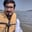Related Tags

python
pandas
row
column
communitycreator

# How to get row number of nth largest value in column in pandasArslan Bajwa

In Python, the pandas library includes built-in functionalities that allow you to perform different tasks with only a few lines of code. One of these functionalities allows you to compute the row number of the nth largest value in a column.

## Representation

### Input

 Row number Column a 0 20 1 5 2 12 3 4 4 1

### Output

Row number of 2nd Largest Value in Column a: 2


## Method

In this method, we first initialize the pandas dataframe. Then we use argsort(), which gives the indices of sorted column of dataframe. Then, we get the row number of the nth largest value from the indices list.

#importing pandas and numpy libraries
import pandas as pd
import numpy as np

#initializing pandas dataframe
df = pd.DataFrame(np.random.randint(1, 100, 20).reshape(10,-1), columns=list('ab'))

#initializing the nth value
n = 5

#computing row number of nth largest value in column "b"
result = df['b'].argsort()[::-1][n]

print(df)

print("Row number in b:", result)

RELATED TAGS

python
pandas
row
column
communitycreator

CONTRIBUTORArslan Bajwa
RELATED COURSES

View all Courses

Keep Exploring

Learn in-demand tech skills in half the time